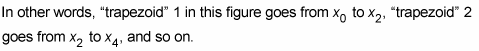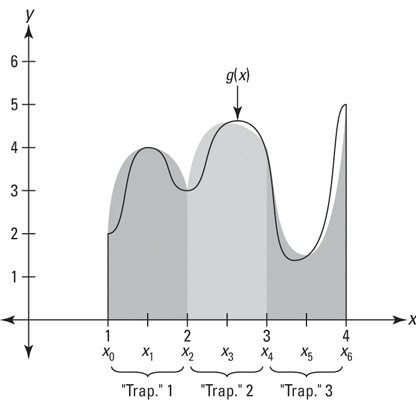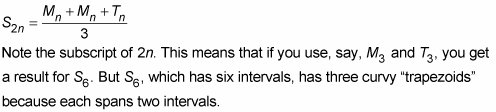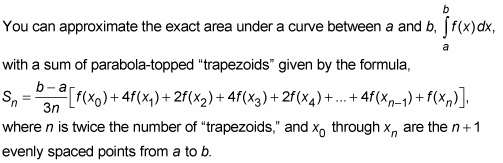##### Differential Equations For DummiesWith Simpson’s rule, you approximate the area under a curve with curvy-topped “trapezoids.” The tops of these shapes are sections of parabolas. You can call them “trapezoids” because they play the same role in Simpson’s rule as the true trapezoids play in the trapezoid rule. Check out three of these curvy-topped shapes in the figure below.

In contrast to the way intervals are used in the left-, right- and midpoint-rectangle rules and in the trapezoid rule, you need two intervals (instead of one) for each “trapezoid” in Simpson's rule.Because of this, the total span must always be divided into an even number of intervals.Simpson’s rule is a very accurate approximation method. In fact, it gives the exact area for any polynomial function of degree three or less. In general, Simpson’s rule gives a much better estimate than either the midpoint rule or the trapezoid rule.

A Simpson’s rule sum or approximation is sort of an average of a midpoint sum and a trapezoid sum, except that you use the midpoint sum twice in the average. So, if you already have the midpoint sum and the trapezoid sum for some number of rectangles or trapezoids, you can obtain the Simpson’s rule approximation with the following simple average:Thus, the above formula always involves the same number of rectangles, trapezoids, and Simpson rule “trapezoids.”

If you don’t have the midpoint and trapezoid sums for the above shortcut, you can use the following formula for Simpson’s rule.